## Appendix II: The Electro-magnetic Field Equations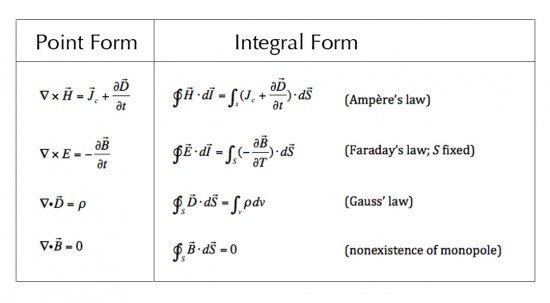Introduction Maxwell’s Equations and the Lorentz Force Law together comprise the e/m field equations; i.e., those equations determining the interactions of charged particles in the vicinity of electric and magnetic fields and the resultant effect of those interactions on the values of the e/m field. For ease of explanation, the…

## Appendix I – Vector Algebra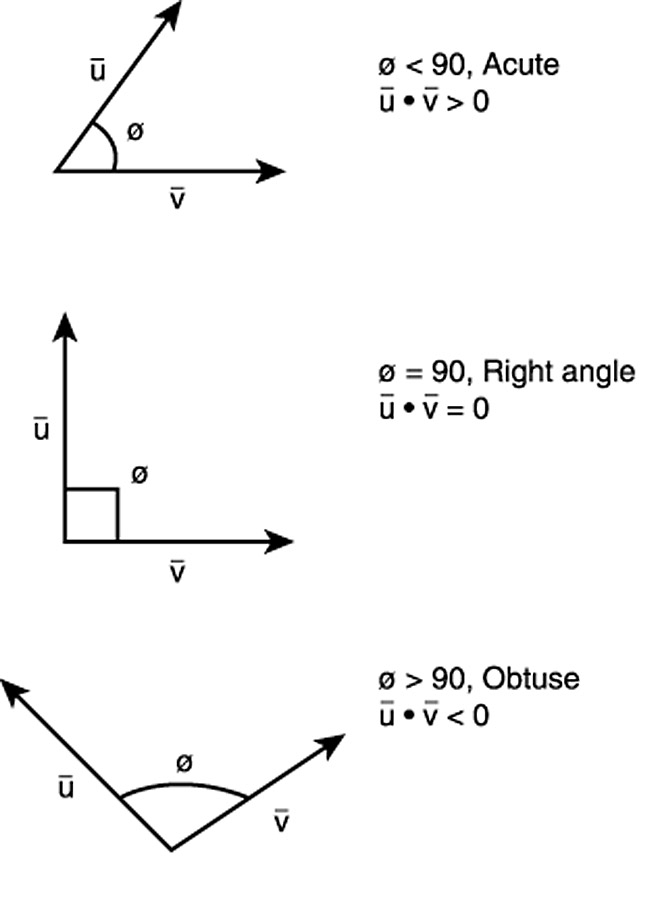Vector algebra is formulated to handle vectors; i.e., quantities with both magnitude and direction. Normal algebra, geometry and trigonometry are efficient at dealing with scalar quantities, that is, those with only magnitude, but are inefficient at handling vectors.  Vector algebra is an efficient way of solving 2D and 3D problems…

## Essential Guide to the EU – Chapter 11Radiation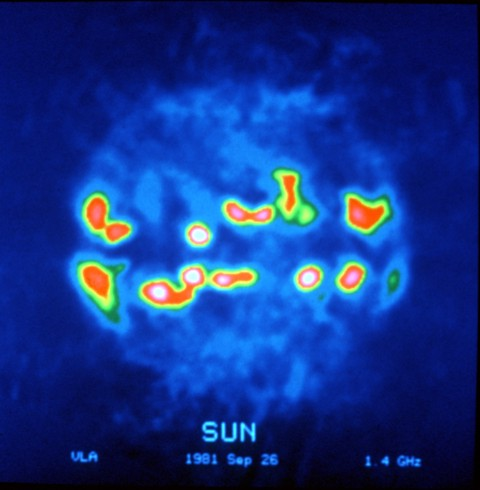11.1  Light Visible light ranges from red through yellow and green to blue and violet. Newton was the first to discover that white light is a mixture of all the colors. White light can be split into its component colors by diffraction through a prism, which ‘bends’ each color by…

## Essential Guide to the EU – Chapter 10Rotational Effects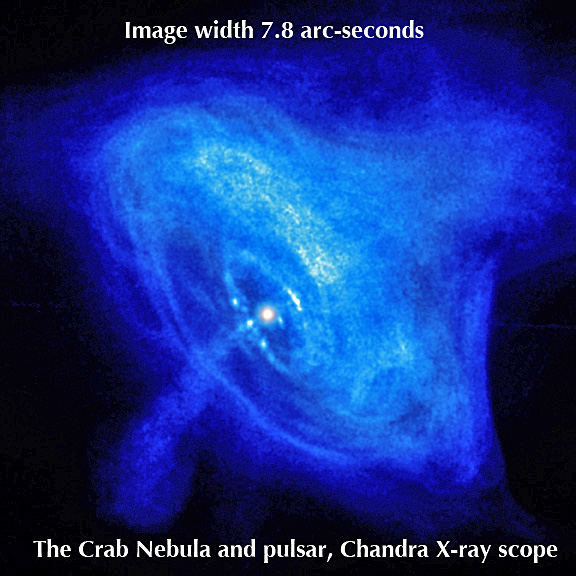10.1  Rotation and Faraday Motors One of the reasons for the assumption of large amounts of Cryogenic (or Cold) Dark Matter (CDM) in the Gravity Model is to explain the observed rotation of galaxies. Astronomers have found that the individual stars in galaxies do not orbit the center of the…

## Essential Guide to the EU – Chapter 9Plasma Circuit Instabilities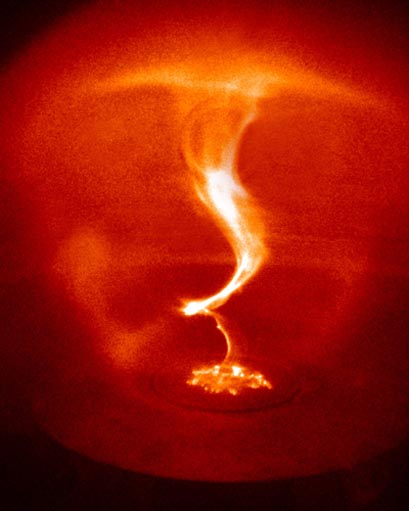9.1  Exploding Double Layers The inductive energy of a circuit is a function of the current and the inductance. If any inductive circuit is disrupted, for example, by opening a switch, then the inductive energy of the circuit will be released at the point of disruption. This is well-known enough…

## Essential Guide to the EU – Chapter 8Current Sheets, Perpendicular Currents and Electric Circuits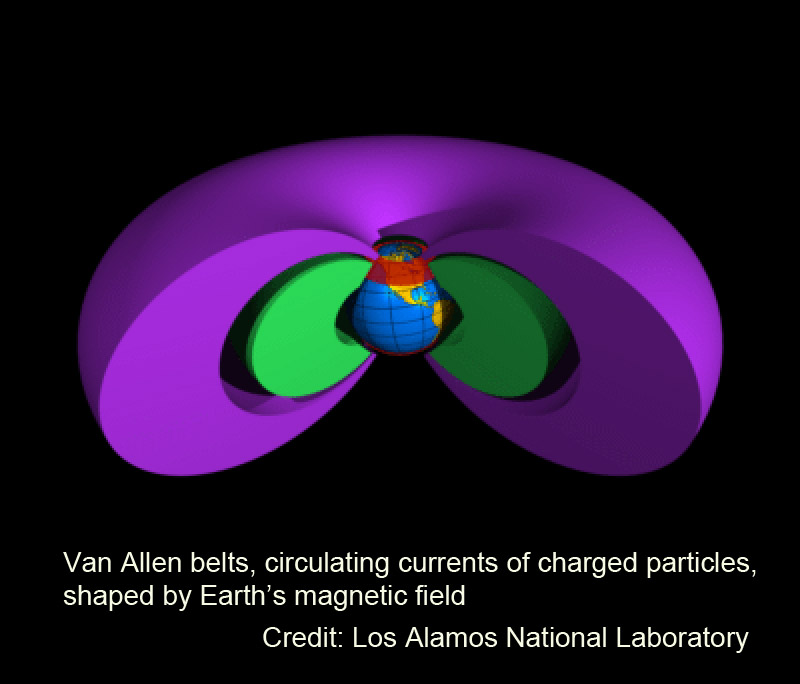8.1  Plasma Current Sheets Reference has already been made to the filamentation of current sheets. This section will explore the nature of current sheets and their relationship to the magnetic field. A current sheet is exactly what it says – a thin surface within which a current flows. It obviously…

## Essential Guide to the EU – Chapter 7 Birkeland Currents, Magnetic Ropes and Current-Carrying Double Layers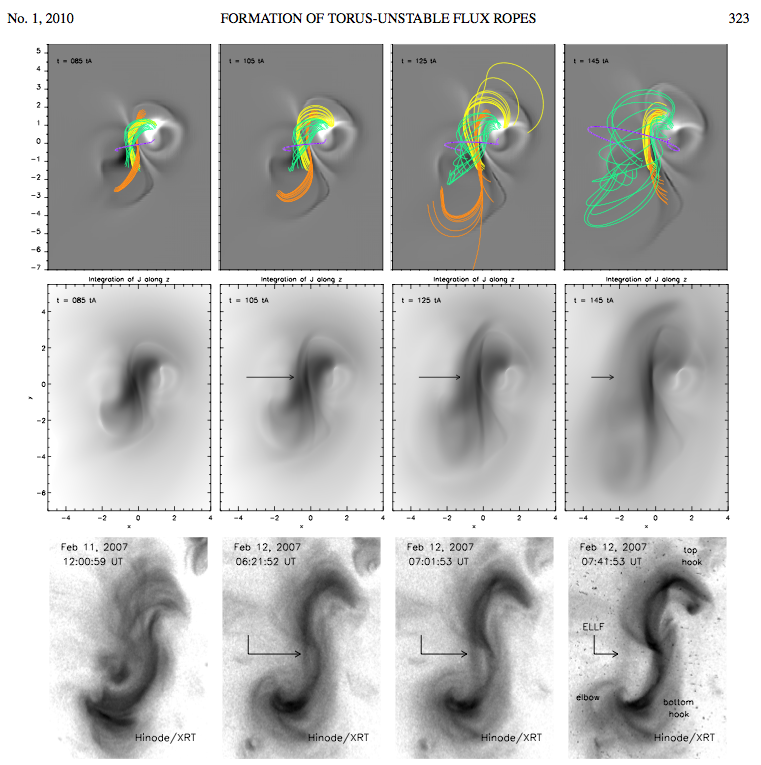7.1 Birkeland Currents There is another cause of filamentation of currents in plasma. This is due to the fact that there is a force of attraction between any two parallel currents. By “parallel currents” we mean that the direction of flow or motion of like charges (say, the electrons, or…

## Essential Guide to the EU – Chapter 6 Currents, Filaments and Pinches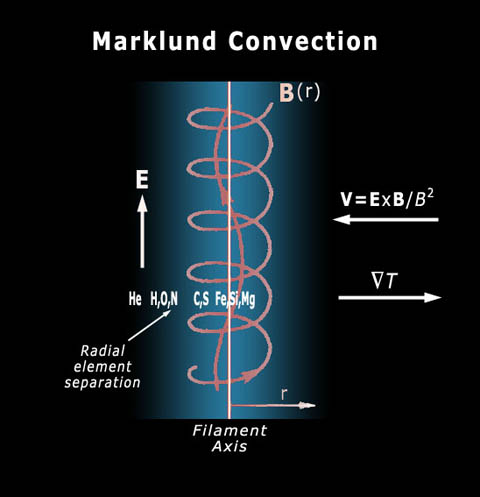6.1  Thermal Motion and Current It is important to distinguish between random thermal motion and uniform linear motion in a plasma. The latter is an electric current which flows due to the presence of an electric field. The random thermal motion is measured by the temperature of the plasma, or…

## Essential Guide to the EU – Chapter 5 Plasma Sheaths, Cells, and Current-Free Double Layers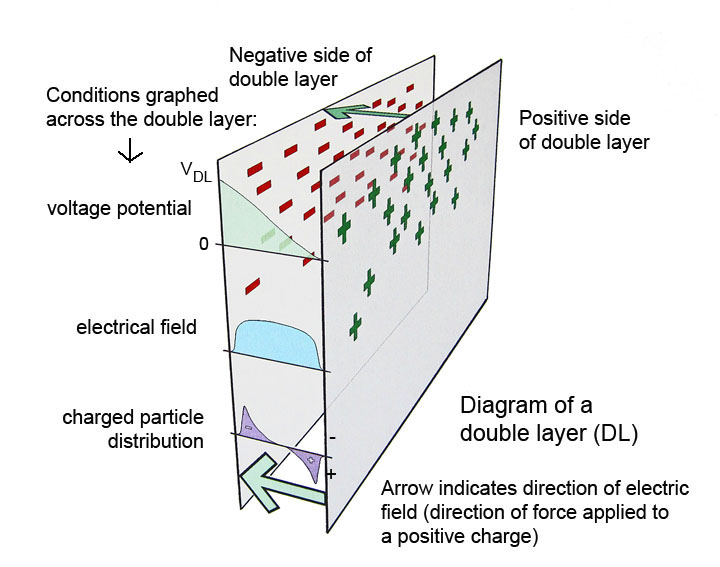5.1  Plasma Temperature and Potential We have seen that temperature is a measure of the thermal energy of the particles in matter. More specifically, temperature is a measure of the kinetic energy of the particles’ random thermal motion. An electron has only 1/1840th the mass (approximately) of a proton, so…

## Essential Guide to the EU – Chapter 4 Electromagnetism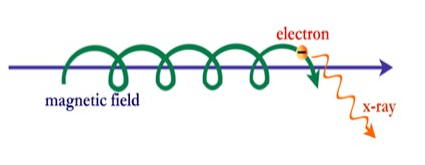4.1  The Electromagnetic Field Equation Scientists attempt to explain physical systems in terms of mathematical models which describe and predict the behavior of the system. For example, Kepler explained the movement of the planets with his three laws. In the same way, plasma behavior is governed by the electromagnetic field…